# 算力 | 手写红黑树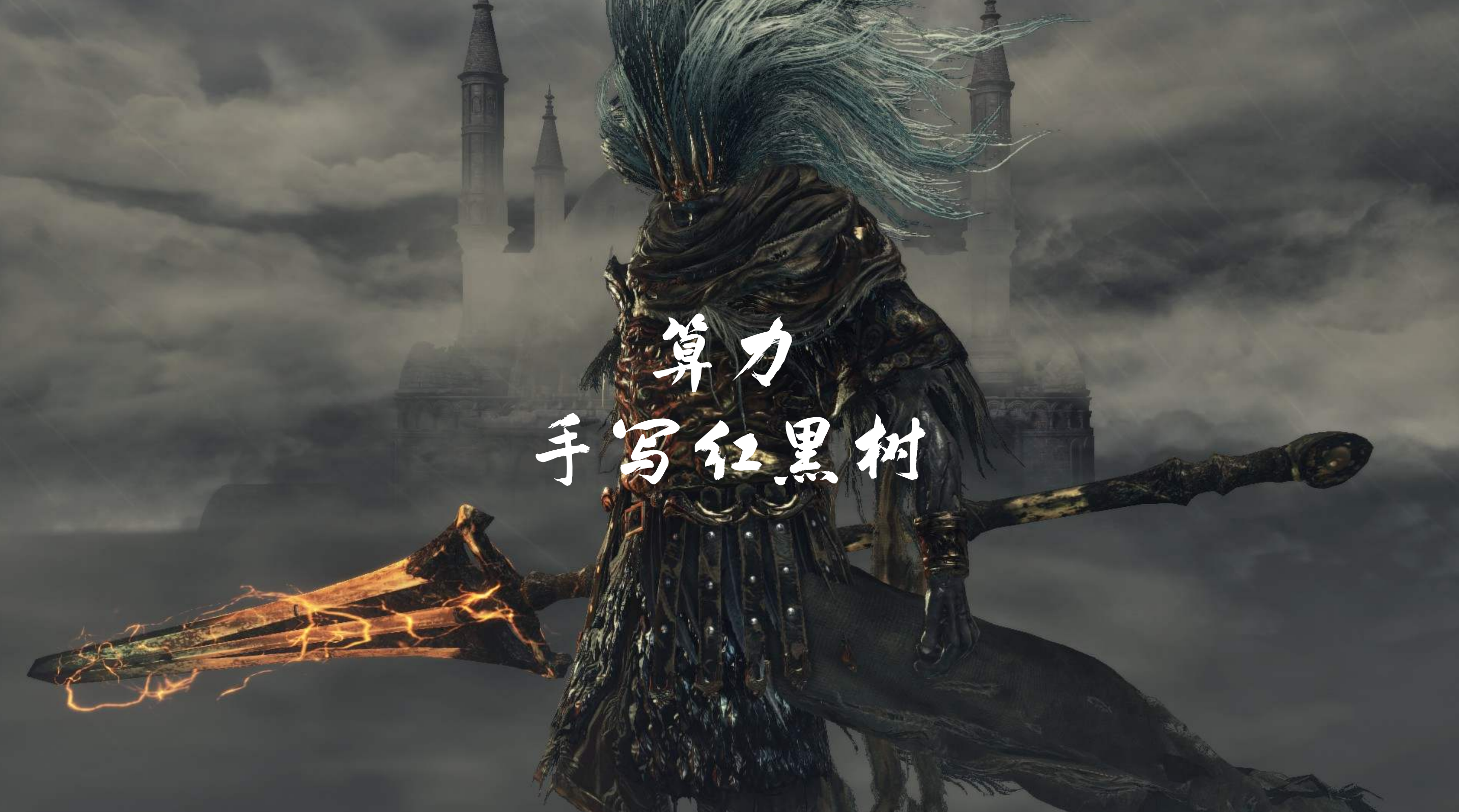### 前世 | 二叉查找树（BS-Tree）

﻿void insert(int key, T value) {    var nn = new Node<>(key, value);    if (Objects.isNull(root)) {        root = nn;    } else {        insert(root, root.parent, nn);    }}void insert(Node<T> n1, Node<T> n2, Node<T> n3) {    if (Objects.isNull(n1)) {        if (n3.key < n2.key) {            n2.left = n3;        } else {            n2.right = n3;        }        n3.parent = n2;    } else {        insert(n3.key < n1.key ? n1.left : n1.right, n1, n3);    }}

﻿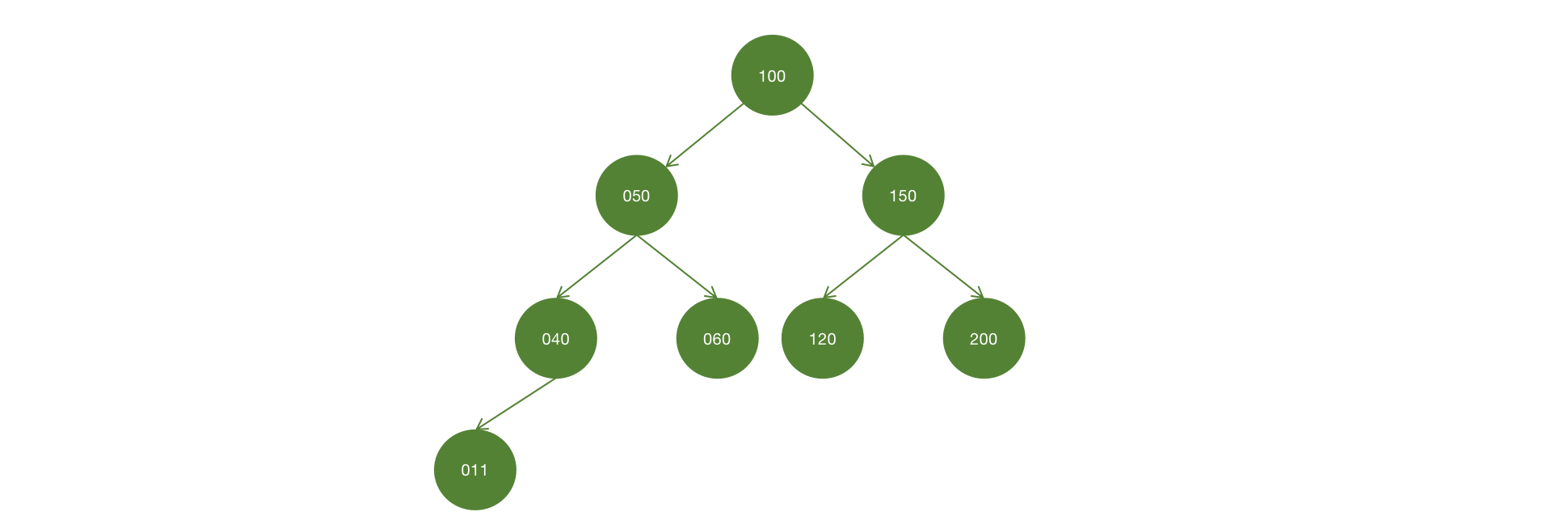void inOrder() {    inOrder(root);}void inOrder(Node<T> n) {    if (Objects.nonNull(n)) {        inOrder(n.left);        System.out.println(n);        inOrder(n.right);    }}

﻿

Node<T> getNode(int key) {    if (Objects.nonNull(root)) {        return root.key == key ? root : getNode(key, root);    }    return null;}Node<T> getNode(int key, Node<T> n) {    if (Objects.nonNull(n)) {        if (key == n.key) {            return n;        }        return getNode(key, key < n.key ? n.left : n.right);    }    return null;}

﻿

• 待删除节点并不存在任何子树；

• 待删除节点仅存在左子树；

• 待删除节点仅存在右子树；

• 待删除节点同时存在左/右子树。

﻿

• 左子树的最右节点；

• 右子树的最左节点。

﻿

Node<T> getSucceedNode(int key) {    var n = getNode(key);    if (Objects.nonNull(n)) {        return getSucceedNode(n.left);//如果左子树不存在任何右节点，后继节点就是左子树    }    return null;}Node<T> getSucceedNode(Node<T> n) {    if (Objects.nonNull(n)) {        var sn = getSucceedNode(n.right);        return Objects.nonNull(sn) ? sn : n;    }    return null;}

﻿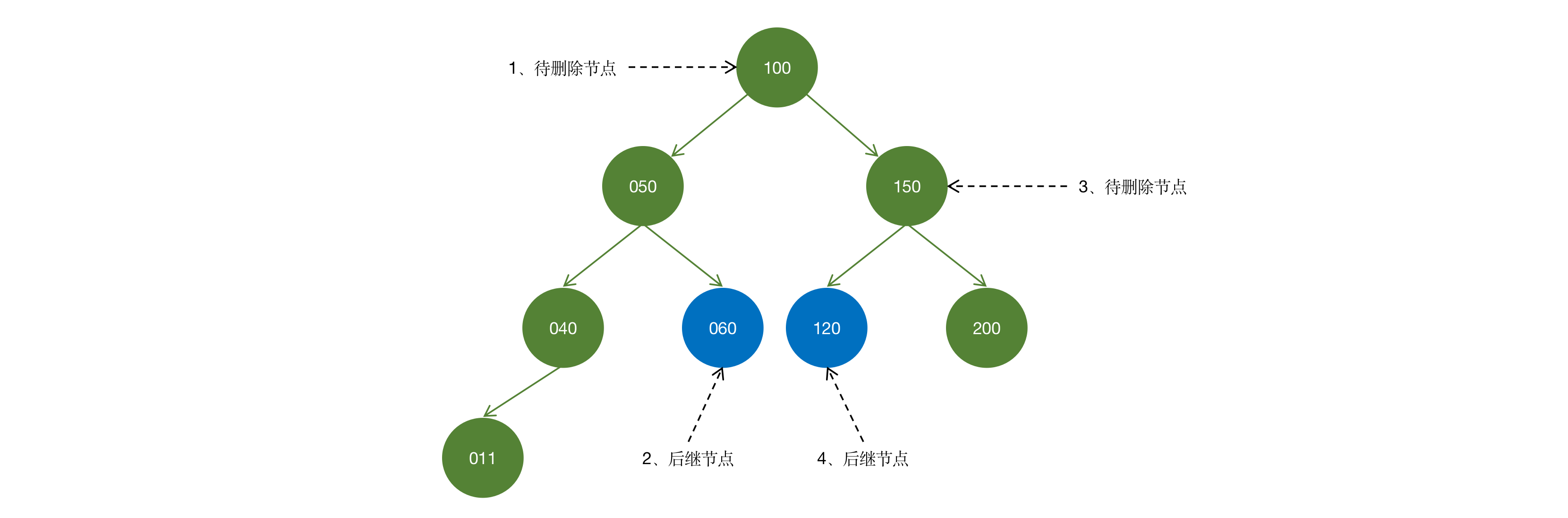boolean delete(int key) {    var n = getNode(key);    if (Objects.nonNull(n)) {        delete(n);        return true;    }    return false;}void delete(Node<T> n) {    if (Objects.nonNull(n.left) && Objects.nonNull(n.right)) {        var sn = getSucceedNode(n.key);        n.key = sn.key;        n.value = sn.value;        n.left.parent = n;        n.right.parent = n;        delete(sn);//递归删除后继节点        return;    } else {        if (Objects.isNull(n.parent)) {            root = Objects.nonNull(n.left) ? n.left : n.right;            root.parent = null;        } else {            var c = Objects.nonNull(n.left) ? n.left : n.right;            if (n.parent.left == n) {                n.parent.left = c;            } else {                n.parent.right = c;            }            if (Objects.nonNull(c)) {                c.parent = n.parent;            }        }    }}

﻿

### 今生 | 红黑树（RB-Tree）if (binCount != 0) {    if (binCount >= TREEIFY_THRESHOLD) //TREEIFY_THRESHOLD 缺省值为8        treeifyBin(tab, i);        if (!added)            return val;        break;}

﻿

• 每个节点，只能是红色或黑色；

• 根节点必须是黑色；

• 如果节点为红色，那么它的左/右子树必须是黑色；

• 任意路径的黑高相同。

﻿### 节点插入修正

﻿

• 插入节点存在uncle节点，且uncle节点为RED；

• 插入节点不存在uncle节点，或uncle节点为BLACK，且在parent的左边；

• 插入节点不存在uncle节点，或uncle节点为BLACK，且在parent的右边。

﻿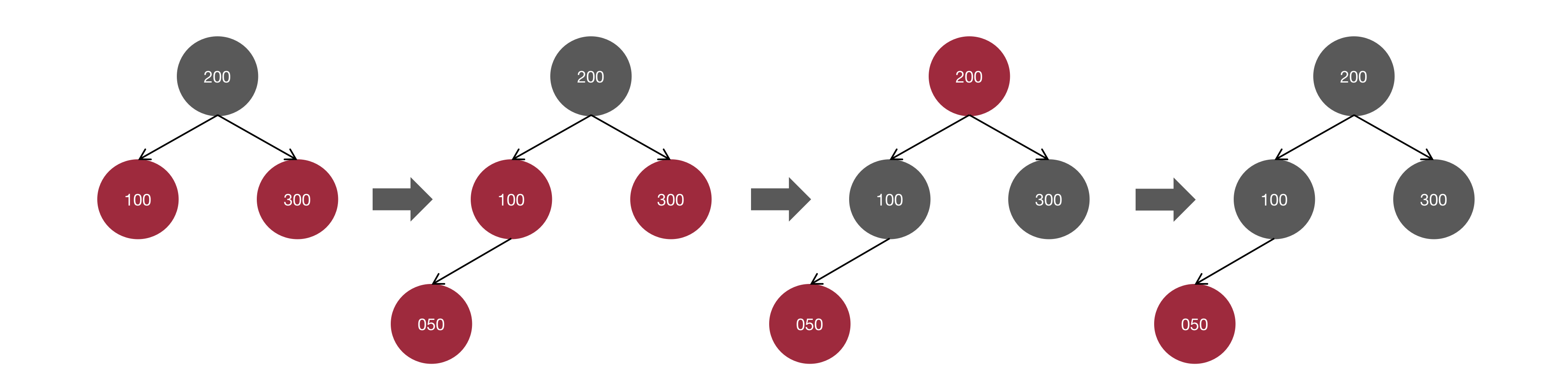void i_fixup(Node<T> n) {    if (root == n) {        root.color = Color.BLACK;//如果是根节点，着色为BLACK并退出        return;    }    if (Objects.isNull(n) || n.parent.color == Color.BLACK) {        return;//如果parent.color为BLACK则退出    } else {            var parent = n.parent;            var gandfather = parent.parent;        if (gandfather.left == parent) {            var uncle = gandfather.right;            if (Objects.nonNull(uncle) && uncle.color == Color.RED) {                parent.color = Color.BLACK;                uncle.color = Color.BLACK;                gandfather.color = Color.RED;                i_fixup(gandfather);//将gandfather节点进行递归修正            }        }    }}

﻿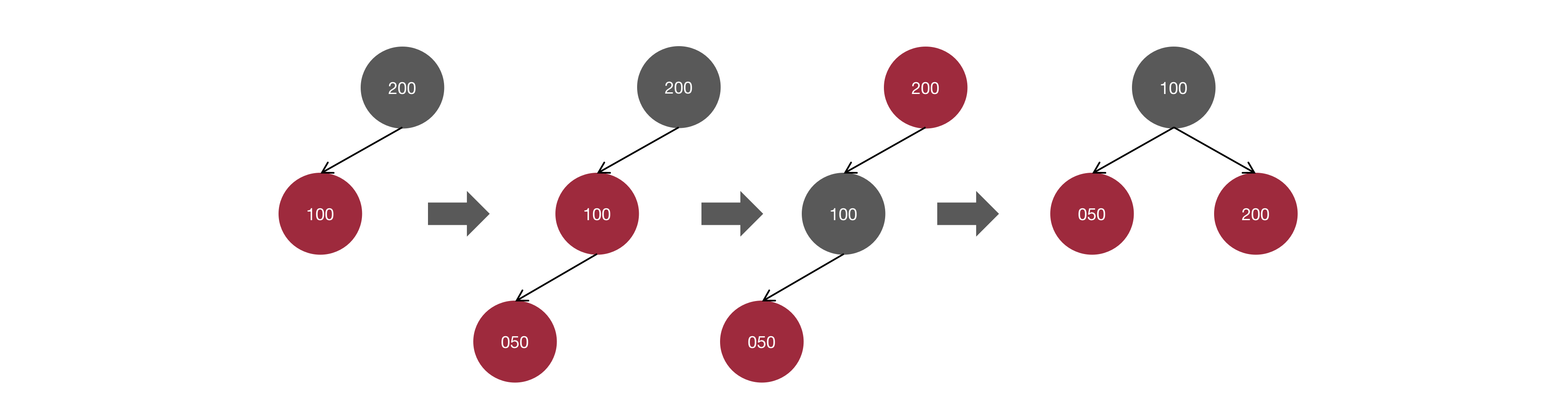if (Objects.nonNull(uncle) && uncle.color == Color.RED) {    //省略情况1相关实现逻辑} else {    if (parent.left == n) {//情况2相关实现逻辑        parent.color = Color.BLACK;        gandfather.color = Color.RED;        rightRotate(gandfather);    }}

﻿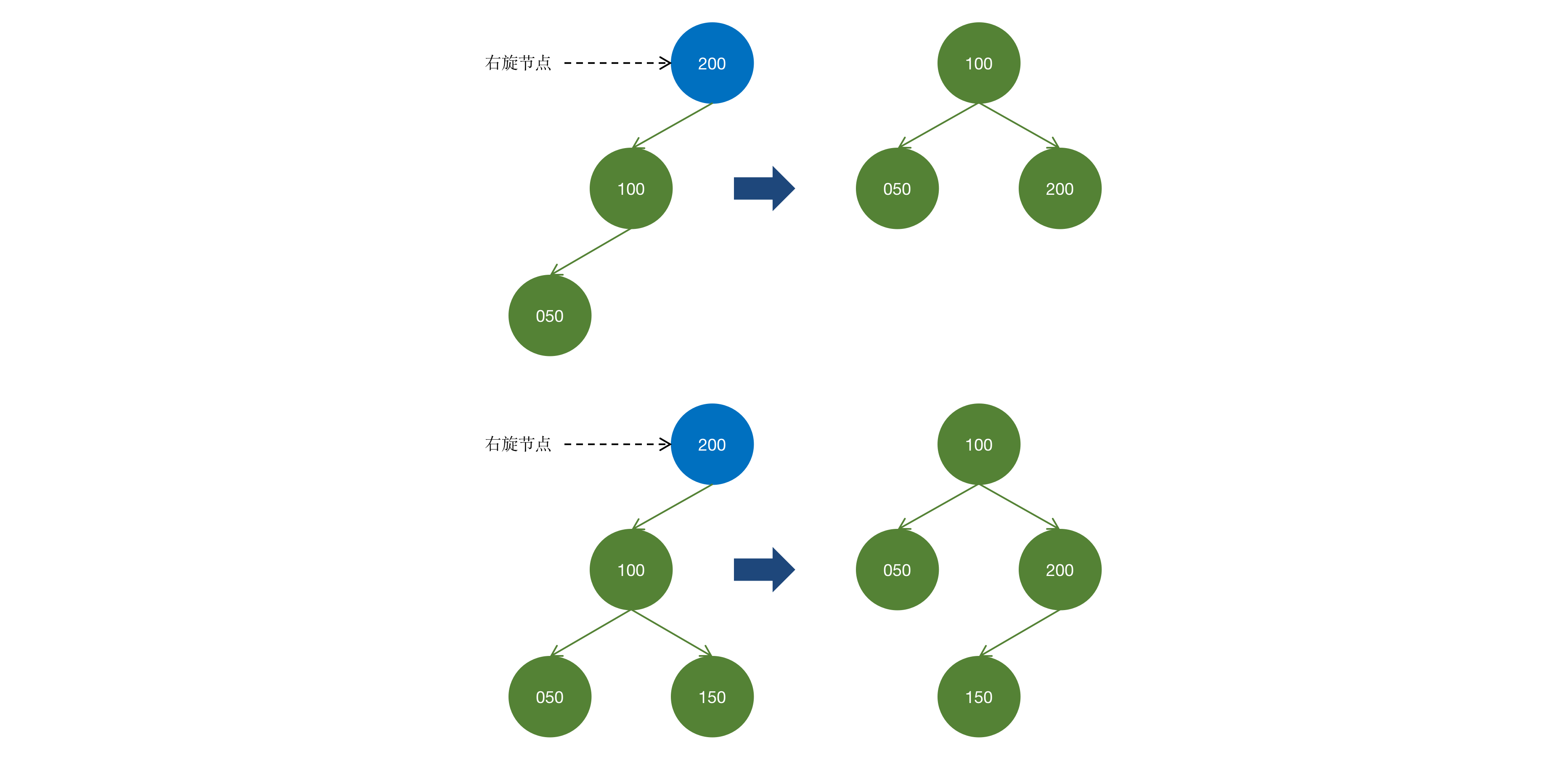void rightRotate(Node<T> n) {    var left = n.left;    n.left = left.right;    if (Objects.nonNull(left.right)) {        left.right.parent = n;    }    left.right = n;    reset(left, n);//重设引用关系}

﻿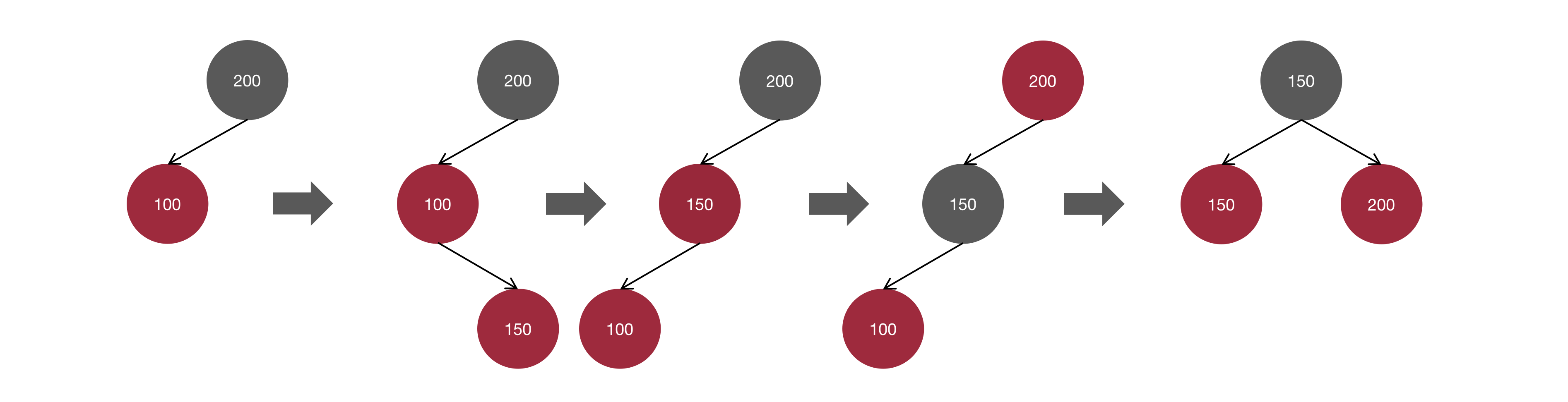if (Objects.nonNull(uncle) && uncle.color == Color.RED) {    //省略情况1实现逻辑} else {    if (parent.left == n) {       //省略情况2实现逻辑    } else {//情况3实现逻辑        leftRotate(parent);        i_fixup(parent);    }}

﻿

### 节点删除修正

• brother节点为RED；

• brother节点为BLACK，且左/右子树为null或BLACK；

• brother节点为BLACK，且存在颜色为RED的左子树；

• brother节点为BLACK，且存在颜色为RED的右子树。

﻿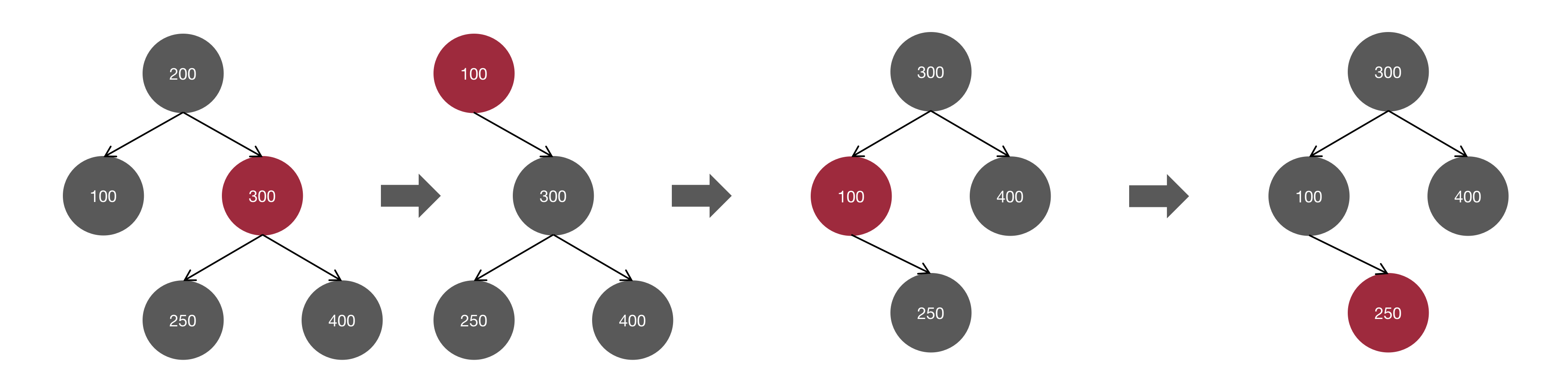void d_fixup(Node<T> n1, Node<T> n2) {    Node<T> bro = null;    while ((Objects.isNull(n1) || n1.color == Color.BLACK) && root != n1) {        if (n2.left == n1) {//情况1相关实现逻辑            bro = n2.right;            if (bro.color == Color.RED) {                bro.color = Color.BLACK;                n2.color = Color.RED;                leftRotate(n2);                bro = n2.right;            }            //情况2相关实现逻辑            if ((Objects.isNull(bro.left) || bro.left.color == Color.BLACK) && (Objects.isNull(bro.right) || bro.right.color == Color.BLACK)) {                if (n2.color == Color.RED) {                    n2.color = Color.BLACK;                    bro.color = Color.RED;                    break;                } else {                    bro.color = Color.RED;                    n1 = n2;                    n2 = n1.parent;                }            }        }    }}

﻿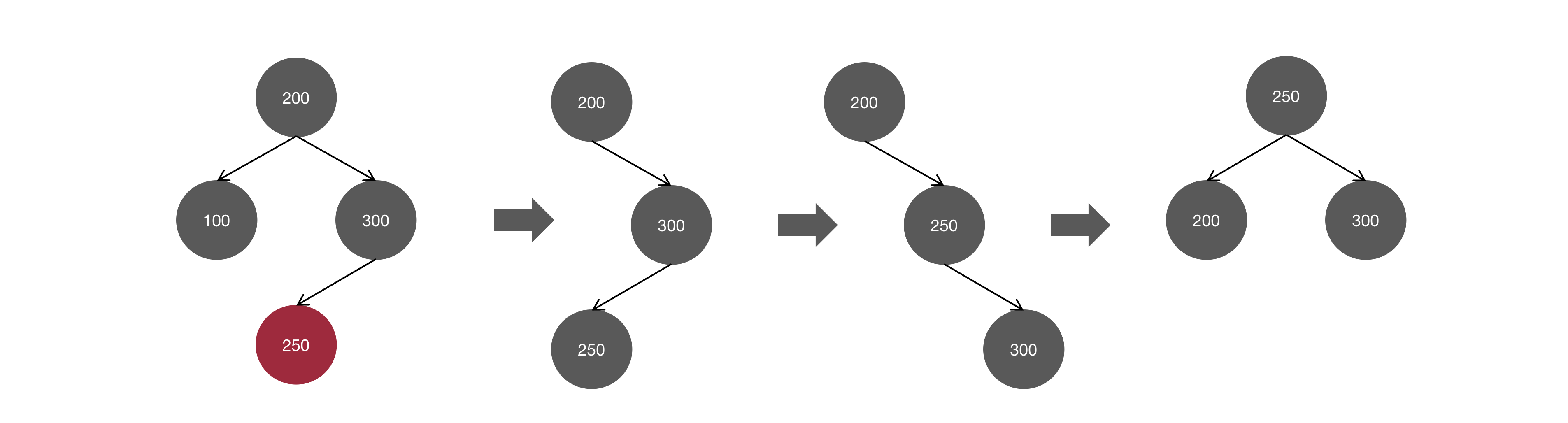if (n2.left == n1) {    bro = n2.right;    if (bro.color == Color.RED) {        //省略情况1相关实现逻辑    }    if ((Objects.isNull(bro.left) || bro.left.color == Color.BLACK) && (Objects.isNull(bro.right) || bro.right.color == Color.BLACK)) {        //省略情况2相关实现逻辑    } else {        if (Objects.nonNull(bro.left) && bro.left.color == Color.RED) {//情况3相关实现逻辑            bro.left.color = n2.color;            n2.color = Color.BLACK;            rightRotate(bro);            leftRotate(n2);        }         break;    }}

﻿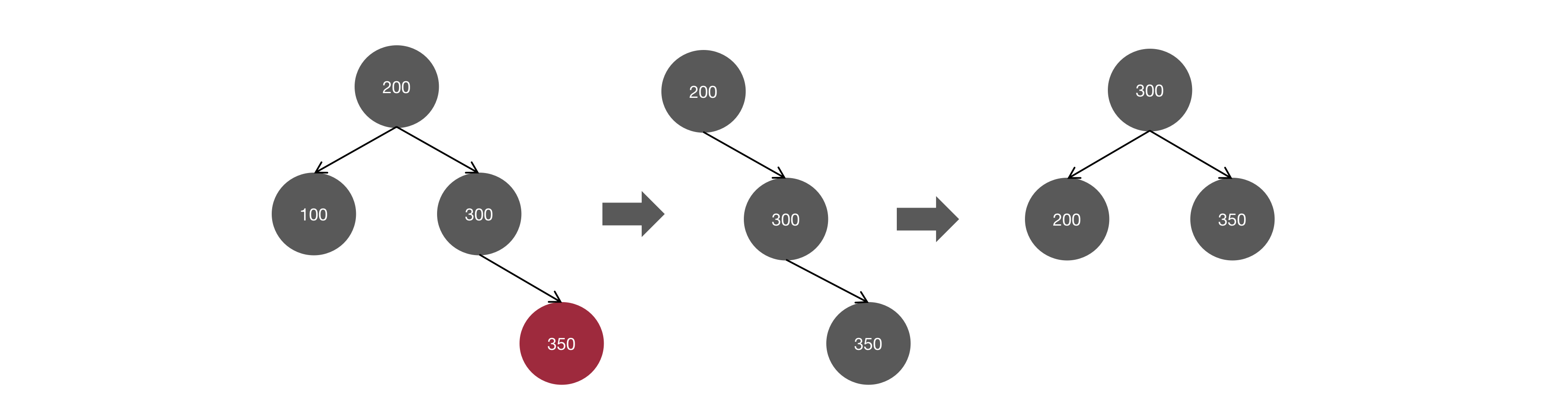if (n2.left == n1) {    bro = n2.right;    if (bro.color == Color.RED) {				//省略情况1相关实现逻辑    }    if ((Objects.isNull(bro.left) || bro.left.color == Color.BLACK) && (Objects.isNull(bro.right) || bro.right.color == Color.BLACK)) {        //省略情况2相关实现逻辑    } else {        if (Objects.nonNull(bro.left) && bro.left.color == Color.RED) {            //省略情况3相关实现逻辑        } else if (Objects.nonNull(bro.right) && bro.right.color == Color.RED) {//情况4相关实现逻辑            bro.color = n2.color;            n2.color = Color.BLACK;            bro.right.color = Color.BLACK;            leftRotate(n2);        }        break;    }}

﻿

﻿

### 码字不易，欢迎转发## 评论